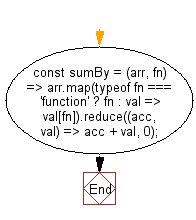# JavaScript: Get the sum of a given array using the provided function

## JavaScript fundamental (ES6 Syntax): Exercise-32 with Solution

Write a JavaScript program to get the sum of a given array, after mapping each element to a value using the provided function.

• Use Array.prototype.map() to map each element to the value returned by fn.
• Use Array.prototype.reduce() to add each value to an accumulator, initialized with a value of 0.

Sample Solution:

JavaScript Code:

``````//#Source https://bit.ly/2neWfJ2
const sumBy = (arr, fn) =>
arr.map(typeof fn === 'function' ? fn : val => val[fn]).reduce((acc, val) => acc + val, 0);

console.log(sumBy([{ n: 4 }, { n: 2 }, { n: 8 }, { n: 6 }], o => o.n));
console.log(sumBy([{ n: -4 }, { n: -2 }, { n: 8 }, { n: 6 }], 'n'));
```
```

Sample Output:

```20
8
```

Pictorial Presentation:Flowchart:Live Demo:

See the Pen javascript-basic-exercise-1-32 by w3resource (@w3resource) on CodePen.

Improve this sample solution and post your code through Disqus

What is the difficulty level of this exercise?

Test your Programming skills with w3resource's quiz.

﻿

## JavaScript: Tips of the Day

Chunks an array into n smaller arrays

Example:

```const tips_chunkIntoN = (arr, n) => {
const size = Math.ceil(arr.length / n);
return Array.from({ length: n }, (v, i) =>
arr.slice(i * size, i * size + size)
);
}
console.log(tips_chunkIntoN([1, 2, 3, 4, 5, 6, 7,8], 4));
```

Output:

```[[1,2],[3,4],[5,6],[7,8]]
```